Image class, variants and internal data augmentation pipeline

## The fastai Image classes¶

The fastai library is built such that the pictures loaded are wrapped in an `Image`. This `Image` contains the array of pixels associated to the picture, but also has a lot of built-in functions that will help the fastai library to process transformations applied to the corresponding image. There are also sub-classes for special types of image-like objects:

See the following sections for documentation of all the details of these classes. But first, let's have a quick look at the main functionality you'll need to know about.

Opening an image and converting to an `Image` object is easily done by using the `open_image` function:

```img = open_image('imgs/cat_example.jpg')
img
```To look at the picture that this `Image` contains, you can also use its `show` method. It will show a resized version and has more options to customize the display.

```img.show()
```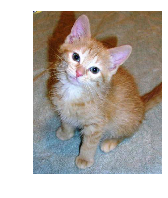This `show` method can take a few arguments (see the documentation of `Image.show` for details) but the two we will use the most in this documentation are:

• `ax` which is the matplolib.pyplot axes on which we want to show the image
• `title` which is an optional title we can give to the image.
```_,axs = plt.subplots(1,4,figsize=(12,4))
for i,ax in enumerate(axs): img.show(ax=ax, title=f'Copy {i+1}')
```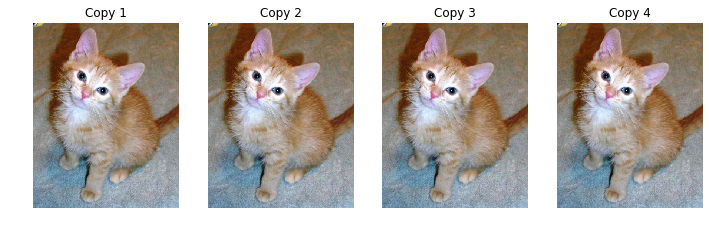If you're interested in the tensor of pixels, it's stored in the `data` attribute of an `Image`.

```img.data.shape
```
`torch.Size([3, 500, 394])`

## The Image classes¶

`Image` is the class that wraps every picture in the fastai library. It is subclassed to create `ImageSegment` and `ImageBBox` when dealing with segmentation and object detection tasks.

### `class``Image`[source][test]

`Image`(`px`:`Tensor`) :: `ItemBase`

Tests found for `Image`:

• `pytest -sv tests/test_vision_transform.py::test_mask_data_aug` [source]

Some other tests where `Image` is used:

• `pytest -sv tests/test_vision_image.py::test_image_resize_same_size_shortcut` [source]

To run tests please refer to this guide.

Support applying transforms to image data in `px`.

Most of the functions of the `Image` class deal with the internal pipeline of transforms, so they are only shown at the end of this page. The easiest way to create one is through the function `open_image`, as we saw before.

#### `open_image`[source][test]

`open_image`(`fn`:`PathOrStr`, `div`:`bool`=`True`, `convert_mode`:`str`=`'RGB'`, `after_open`:`Callable`=`None`) → `Image`

No tests found for `open_image`. To contribute a test please refer to this guide and this discussion.

Return `Image` object created from image in file `fn`.

If `div=True`, pixel values are divided by 255. to become floats between 0. and 1. `convert_mode` is passed to `PIL.Image.convert`.

With the following example, you can get a feel of how `open_image` working with different `convert_mode`. For all the modes see the source here.

```from fastai.vision import *
```
```path_data = untar_data(URLs.PLANET_TINY); path_data.ls()
```
```[PosixPath('/Users/Natsume/.fastai/data/planet_tiny/labels.csv'),
PosixPath('/Users/Natsume/.fastai/data/planet_tiny/train')]```
```il = ImageList.from_folder(path_data/'train'); il
```
```ImageList (200 items)
Image (3, 128, 128),Image (3, 128, 128),Image (3, 128, 128),Image (3, 128, 128),Image (3, 128, 128)
Path: /Users/Natsume/.fastai/data/planet_tiny/train```
```il.convert_mode = 'L'
il.open(il.items)
```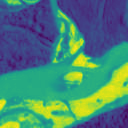```mode = '1'
open_image(il.items,convert_mode=mode)
```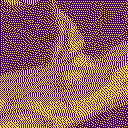As we saw, in a Jupyter Notebook, the representation of an `Image` is its underlying picture (shown to its full size). On top of containing the tensor of pixels of the image (and automatically doing the conversion after decoding the image), this class contains various methods for the implementation of transforms. The `Image.show` method also allows to pass more arguments:

#### `Image.show`[source][test]

`Image.show`(`ax`:`Axes`=`None`, `figsize`:`tuple`=`(3, 3)`, `title`:`Optional`[`str`]=`None`, `hide_axis`:`bool`=`True`, `cmap`:`str`=`None`, `y`:`Any`=`None`, **`kwargs`)

No tests found for `show`. To contribute a test please refer to this guide and this discussion.

Show image on `ax` with `title`, using `cmap` if single-channel, overlaid with optional `y`

• ax: matplotlib.pyplot axes on which show the image
• figsize: Size of the figure
• title: Title to display on top of the graph
• hide_axis: If True, the axis of the graph are hidden
• cmap: Color map to use
• y: Potential target to be superposed on the same graph (mask, bounding box, points)

This allows us to completely customize the display of an `Image`. We'll see examples of the `y` functionality below with segmentation and bounding boxes tasks, for now here is an example using the other features.

```img.show(figsize=(2, 1), title='Little kitten')
``````img.show(figsize=(10,5), title='Big kitten')
```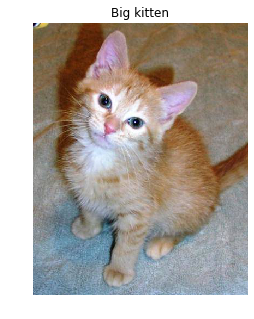With the following example, you will get a feel of how to set `cmap` for `Image.show`.

See matplotlib docs for `cmap` options here. This is how `defaults.cmap` is defined in `fastai`, see source here.

```defaults = SimpleNamespace(cpus=_default_cpus, cmap='viridis', return_fig=False, silent=False)
```
```img.shape
```
`torch.Size([3, 500, 394])`

As `cmap` works on a single channel, so it is necessary to set `convert_mode='L'` so that the image channel will be shrinked to 1.

```img = open_image('imgs/cat_example.jpg', convert_mode='L'); print(img.shape)
img
```
```torch.Size([1, 500, 394])
```